1. Home
2. Docs
3. Numerical Methods
4. Solution of Nonlinear Equations
5. Errors in Computing

# Errors in Computing

## True Error

True error is denoted by Et and is defined as the difference between the true value ad approximate value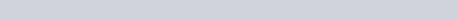## Relative Error

Relative error is denoted by Er, and is defined as the ratio between the true error and the true value.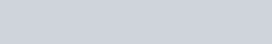## Approximate Error

Approximate error is denoted by Ea, and is defined as the difference between the present approximation and previous approximation.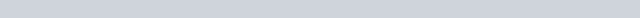## Relative Approximate Errors

Relative approximate error is denoted by Em, and is defined as the ratio between the approximate error and the present approximation.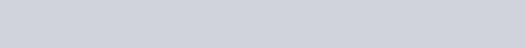## Sources of Error

### Turncation Errors:

Turncation errors arises from using an approximation in place of exact mathematical procedure. It is the error resulting from the truncation of the numerical process. We often use some finite number of terms to estimate the sum of a finite series.

For e.g.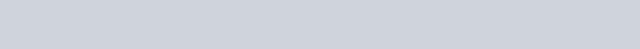### Round off Errors:

Round off errors occurs when fixed number of digits are used to represent exact number. Since, the numbers are stored at every stage of computation; round off errors is introduced at the end of every arithmetic operation.